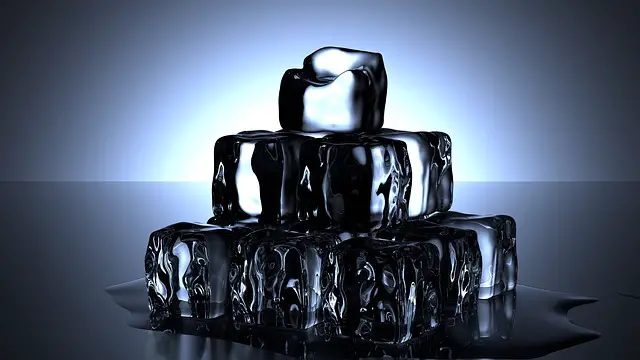# Density & Relative density

Last updated on May 16th, 2022 at 08:34 pm

Density is the mass per unit volume. The unit of density in SI is Kg/meter3. The relative density or specific gravity of a substance is the ratio of its own density and the density of water. It’s a number only without any unit.

## What is the difference between density and relative density?

Density is the mass per unit volume. So if we divide the mass of a substance by its volume then we get the density of that substance. The unit of density in SI is Kg/meter3.
The relative density or specific gravity of a substance is the ratio of its own density and the density of water. It’s a comparative parameter calculated with respect to water’s density. It’s a ratio of two densities and a number only without any unit.

## Unit of density (in SI and CGS)

### What is the unit of density in SI?

The unit of density in SI is Kg/meter3.

### What is the unit of density in CGS?

The unit of density in SI is gm/centimeter3.

## Density chart – with density values of selected substances

The density of different substances in SI and CGS are listed below in the diagram (density values of air, wood, petrol, ice, water, concrete, glass, granite, aluminium, steel (stainless), copper, lead, mercury, gold, platinum, osmium, expanded polystyrene)

### What is the density of ice in SI?

The density of ice is 916 kg/meter3 in SI. This means if we take a slab of ice of unit volume (length=1 meter, breadth = 1 meter and height = 1 meter), and measure its mass then its mass will be 916 kg.

### What is the density of water in SI?

The density of water is 1000 kg/m3 in SI. This means water of 1 cubic meter volume has a mass of 1000 kg. In CGS the density of water is 1 gm/cm3.

## Density Equation | density calculation using formula

The density equation is written in this way: ρ = m/V, where ρ is the symbol of density, m is mass and V represents volume.

We get the equation of volume: V=m/ρ

We also get an equation of mass: m=ρV

## What is Relative Density?

The Relative density or specific gravity of a substance is defined as the ratio of its own density and the density of water at 4 °C.
It’s a number only without any unit.Relative density of ice = density of ice / density of water = 916/1000 = 0.916

### What is the relative density of water?

The relative density of water is certainly 1.
[Relative density of water = density of water/density of water = 1]

### What is the relative density of ice?

Relative density of ice = density of ice / density of water = 916/1000 = 0.916

### How does an ice cube float in water?

A substance with relative density greater than 1 will sink in water and a substance with relative density less than 1 will float in water.
The relative density of ice is only 0.916. So we can say an ice cube will float in water. In other words, Ice has less density than water, hence it experiences an upthrust applied by water which is more than the weight of ice. Hence an ice cube floats in water.

## Relative Density Units

### What is the CGS unit of relative density?

It’s a number only without any unit.

### What is the SI unit of relative density?

It’s a number only without any unit.

## Why is the density of water used as the reference to measure relative density?

We calculate relative density with respect to water. Why? Generally, the density of water at 4 °C (the temperature at which the density of water reaches its maximum value) is used as the reference to measure relative density.

In SI units, the density of water is approximately 1000 kg/m3 and in CGS units it’s 1 g/cm3 which makes relative density calculations pretty convenient.

The density of the material only needs to be divided by 1000 or 1, depending on the units.

## Relative density Examples

Here are some samples of relative density.
Water: 1
Iron: 7.87
Table Salt: 2.17
Mercury:13.56

Suggested Study: Mole vs Molecule